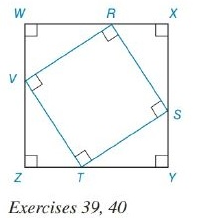Chapter 8.2, Problem 40E### Elementary Geometry for College St...

6th Edition
Daniel C. Alexander + 1 other
ISBN: 9781285195698

#### Solutions

Chapter
Section### Elementary Geometry for College St...

6th Edition
Daniel C. Alexander + 1 other
ISBN: 9781285195698
Textbook Problem
1 views

# Square RSTV is inscribed in square WXYZ, as shown. If Z T = 8 and T Y = 15 , Finda) the perimeter of RSTVb) the area of RSTVTo determine

To Find:

a. The perimeter of the square RSTV.

b. The area of the square RSTV.

Explanation

Formula Used:

1. Pythagorean theorem for the right angle triangle ABC for the hypotenuse AC,

AC2=AB2+BC2.

2. The perimeter of a polygon is the sum of the lengths of all sides of the polygon. P=4s, where s is side length of the square.

3. Area of the square =s2, where s is side length of the square.

It is given that the square RSTV is inscribed in square WXYZ with ZT=8 and TY=15.

The side ZY is split into ZT and TY with dimension 8 and 15 respectively.

As WXYZ is a square, each side of RSTV can also be split into two parts. That is, 8 and 15 respectively. Therefore, ZT=YS=XR=WV=8 and TY=SX=RW=VZ=15

### Still sussing out bartleby?

Check out a sample textbook solution.

See a sample solution

#### The Solution to Your Study Problems

Bartleby provides explanations to thousands of textbook problems written by our experts, many with advanced degrees!

Get Started

#### Evaluate the integrals in Problems 1-26.

Mathematical Applications for the Management, Life, and Social Sciences

#### In Exercises 107-120, factor each expression completely. 116. (x + y)2 1

Applied Calculus for the Managerial, Life, and Social Sciences: A Brief Approach

#### The polar form for the graph at the right is:

Study Guide for Stewart's Single Variable Calculus: Early Transcendentals, 8th